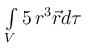Courses

# Vector Calculus MCQ Level - 2

## 10 Questions MCQ Test Topic wise Tests for IIT JAM Physics | Vector Calculus MCQ Level - 2

Description
This mock test of Vector Calculus MCQ Level - 2 for IIT JAM helps you for every IIT JAM entrance exam. This contains 10 Multiple Choice Questions for IIT JAM Vector Calculus MCQ Level - 2 (mcq) to study with solutions a complete question bank. The solved questions answers in this Vector Calculus MCQ Level - 2 quiz give you a good mix of easy questions and tough questions. IIT JAM students definitely take this Vector Calculus MCQ Level - 2 exercise for a better result in the exam. You can find other Vector Calculus MCQ Level - 2 extra questions, long questions & short questions for IIT JAM on EduRev as well by searching above.
QUESTION: 1

### The line integral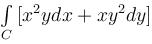taken along the closed path formed by y = x and x2 = y3 in the first quadrant will be valuated to :

Solution:

By Green's theorem, we have,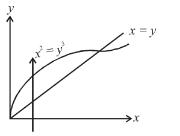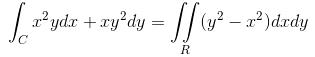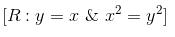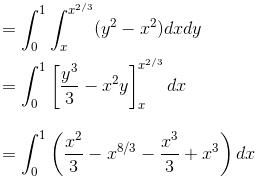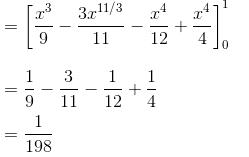QUESTION: 2

###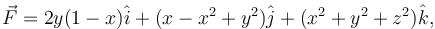where S : x2 + y2 + z2 = 1, z ≥ 0 and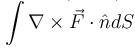will have the value :

Solution:

By Stoke’s Theorem,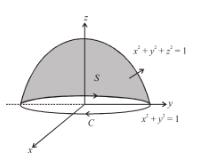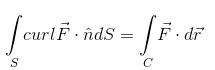where C : x2 + y2 = 1, z = 0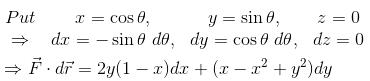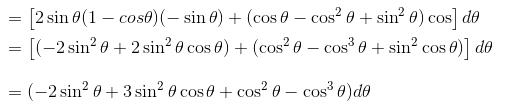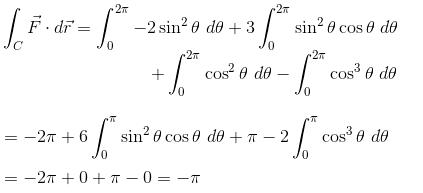QUESTION: 3

### The line integral of the vector field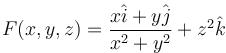along the helix defined by x = cos t, y = sin t,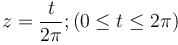is equal to :

Solution:

We have,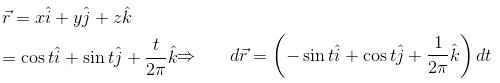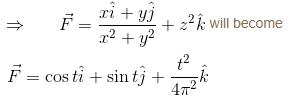Hence, the line integral of the given vector field will be,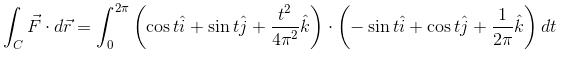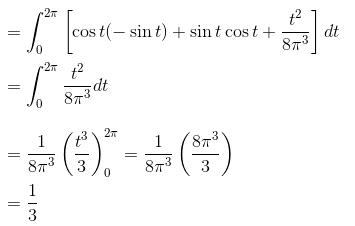QUESTION: 4

If  u = x + y + z, v = x2 + y2 + z2  and w = yz = zx + xy then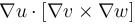is equal to :

Solution:

u = x + y + z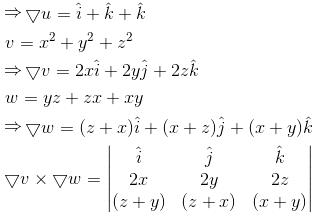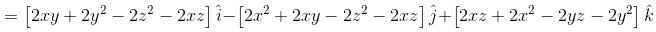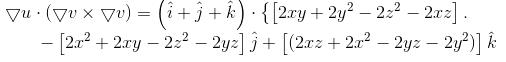= 0

QUESTION: 5

The potential function for the vector field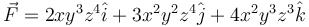will be :

Solution:

Compare the given vector field with the gradient of some function, say φ, i.e.,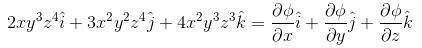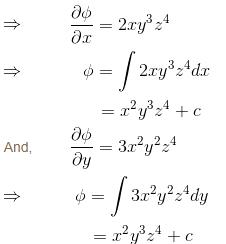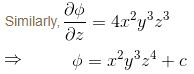⇒ The potential function for the given vector field would be x2y3z4.

QUESTION: 6

The value of the surface integral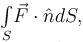where S is the closed surface of the solid bounded by the graphs of x = 4 and z = 9 – y2 and coordinate planes &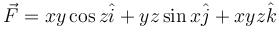will be given by :

Solution:

The given surface is z + y2 = 9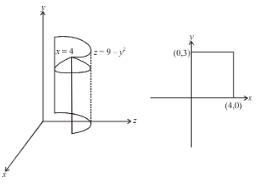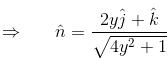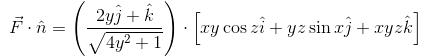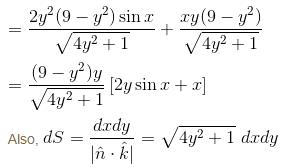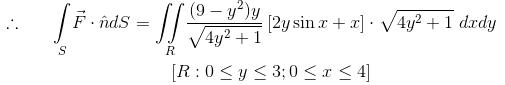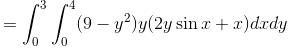The correct answer is: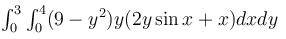QUESTION: 7

The value of the integral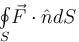where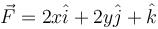and where S is the entire surface of the  paraboloid z = 1 - x2 - y2 with z = 0 together with the disk {(x, y) : x2 + y2 < 1}

Solution: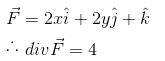By Gauss Divergence Theorem,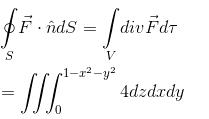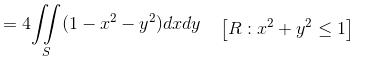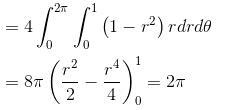QUESTION: 8

The value of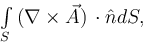where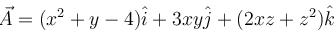and S is the surface of the paraboloid z = 4 – (x2 + y2) above the xy-plane will be :

Solution:

By Stokes theorem,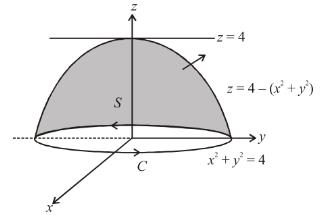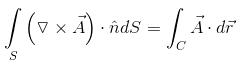Here, the boundary curve C of the surface will be given by x2 + y2 = 4, z = 0, or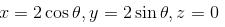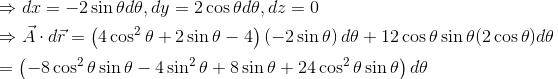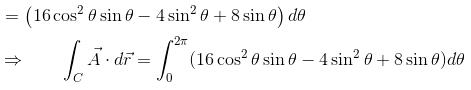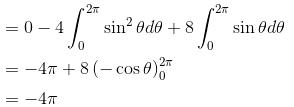QUESTION: 9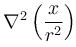is equal to :

Solution:

Consider,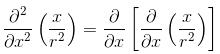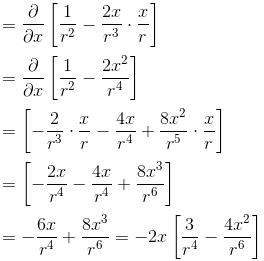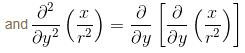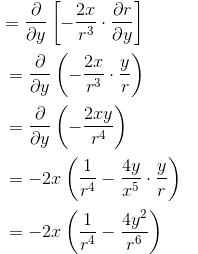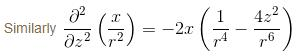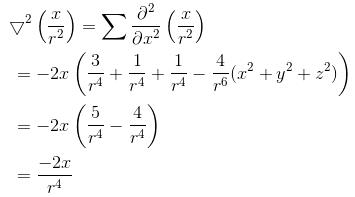The correct answer is: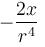QUESTION: 10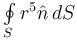is equivalent to :

Solution: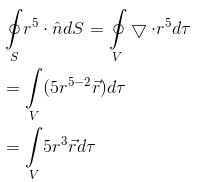The correct answer is: